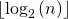Practice Questions for Recursion | Set 2

• Difficulty Level : Easy
• Last Updated : 13 Jul, 2021

Explain the functionality of following functions.

Question 1

C

 /* Assume that n is greater than or equal to 1 */int fun1(int n){    if (n == 1)        return 0;    else        return 1 + fun1(n / 2);}

Java

 /* Assume that n is greater than or equal to 1 */static int fun1(int n){    if (n == 1)        return 0;    else        return 1 + fun1(n / 2);} // This code is contributed by shubhamsingh10

Python3

 # Assume that n is greater than or equal to 1 */def fun1(n):    if(n == 1):        return 0    else:        return 1 + fun1(n//2) # This code is contributed by shubhamsingh10

C#

 /* Assume that n is greater than or equal to 1 */static int fun1(int n){    if (n == 1)        return 0;    else        return 1 + fun1(n / 2);} // This code is contributed by shubhamsingh10



C++

 /* Assume that n is greater than or equal to 1 */int fun1(int n){    if (n == 1)        return 0;    else        return 1 + fun1(n / 2);} // This code is contributed by shubhamsingh10// Improved by Adwitiya Mourya

Answer: The function calculates and returns. For example, if n is between 8 and 15 then fun1() returns 3. If n is between 16 to 31 then fun1() returns 4.
Question 2

C++

 /* Assume that n is greater than or equal to 0 */void fun2(int n){if(n == 0)    return; fun2(n/2);cout << n%2 << endl;} //This code is contributed by shubhamsingh10

C

 /* Assume that n is greater than or equal to 0 */void fun2(int n){  if(n == 0)    return;   fun2(n/2);  printf("%d", n%2);}

Java

 /* Assume that n is greater than or equal to 1 */static void fun2(int n){if(n == 0)    return;  fun2(n/2);System.out.println(n%2);} // This code is contributed by Shubhamsingh10

Python3

 # Assume that n is greater than or equal to 0 */def fun2(n):    if(n == 0):        return         fun2(n / 2)    print(n % 2, end="") # This code is contributed by shubhamsingh10

C#

 void fun2(int n){if(n == 0)    return;   fun2(n/2);Console.Write(n%2);}// This code is contributed by shubhamsingh10

Javascript



Answer: The function fun2() prints binary equivalent of n. For example, if n is 21 then fun2() prints 10101.
Note that above functions are just for practicing recursion, they are not the ideal implementation of the functionality they provide.